## 三角函数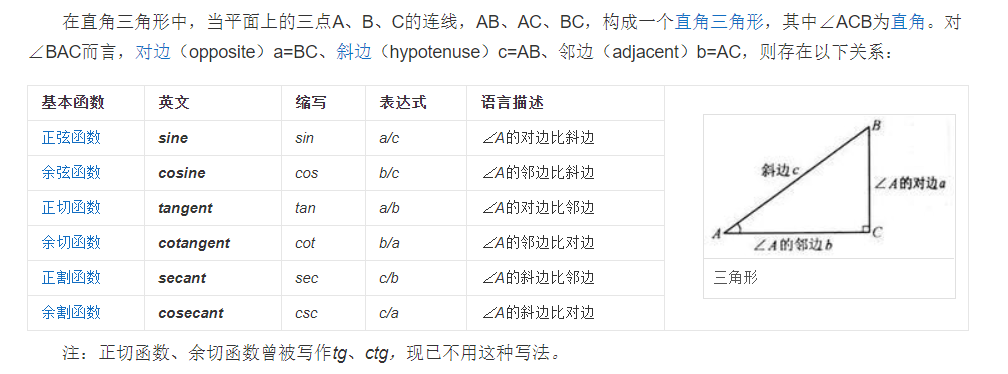### 特殊角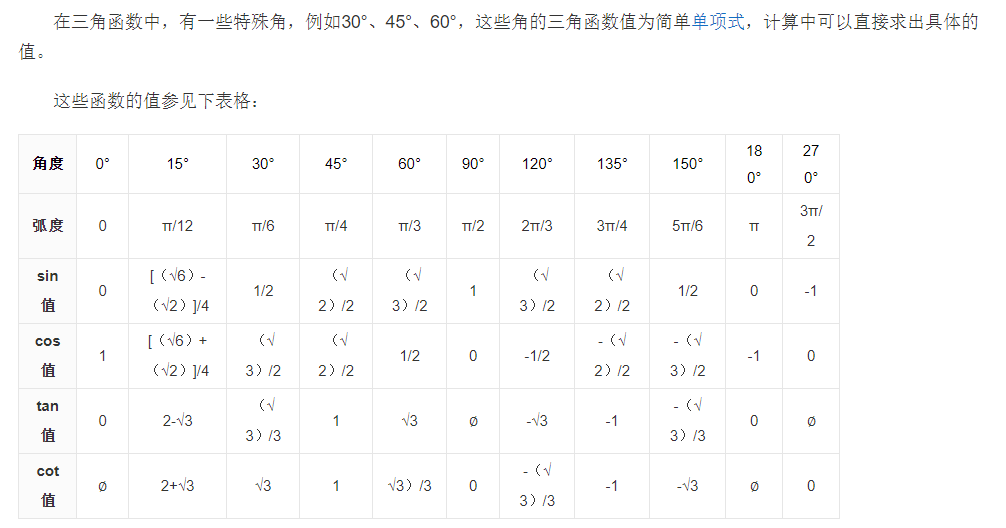### 几何性质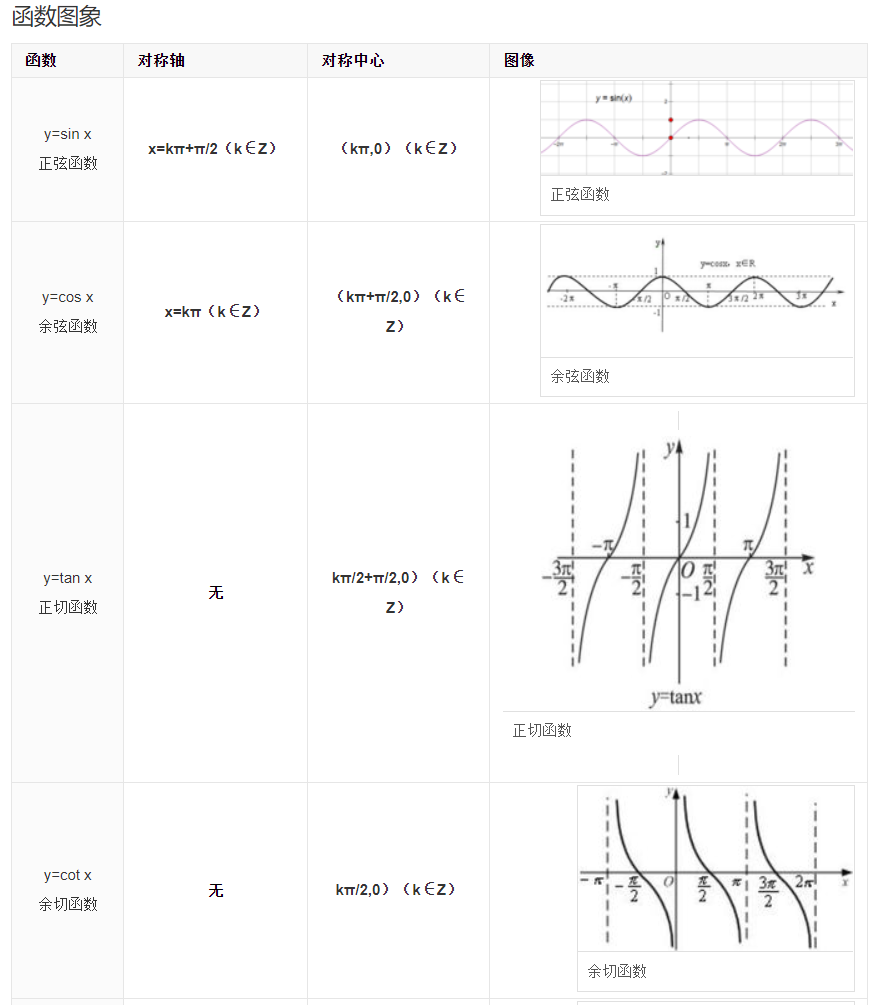### 公式

sin（2kπ+α）=sin α sin（π+α）=-sin α
cos（2kπ+α）=cos α cos（π+α）=-cos α
tan（2kπ+α）=tan α tan（π+α）=tan α
cot（2kπ+α）=cot α cot（π+α）=cot α
sec（2kπ+α）=sec α sec（π+α）=-sec α
csc（2kπ+α）=csc α csc（π+α）=-csc α

sin（-α）=-sin α sin（π-α）=sin α
cos（-α）=cos α cos（π-α）=-cos α
tan（-α）=-tan α tan（π-α）=-tan α
cot（-α）=-cot α cot（π-α）=-cot α
sec（-α）=sec α sec（π-α）=-sec α
csc（-α）=-csc α csc（π-α）=csc α

## UE4的三角函数

• Degree就是用的基础的直角三角形函数,例如Sine是` Sinθ=a/c`计算，输入参数是角度，得到的是[0,1]

Sine(PI)–>0

Sine(PI/2)–>1

ASine(1)–>PI/2

ASine(-1)–>-PI/2

SineD(30)–>0.5

SineD(90)–>1

ASineD(0.5)–>30

ASineD(1)–>90

### Cos,ACos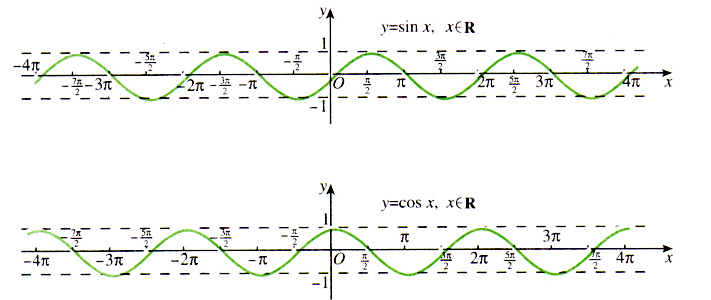Cos(PI)–>-1

ACos(1)–>0

ACos(-1)–>PI

Cos(60)–>0.5

Cos(0)–>1

### Tan

• 蓝图没有提供Cot方法，本质上就是tan的2个参数反转一下
##### 特殊角度的值

Tan(PI)–>0

Tan(PI/2) –>垃圾值，如图

ATan(0)–>0

ATan(无穷)–>接近于PI/2,如图

TanD(45)–>1

TanD(90)–>垃圾值，因为分母=0

ATanD(1)–>45

ATanD(0)–>0

ATanD( 无穷大)–>接近于90

##### 蓝图额外Tan方法

ATan2有2个方法，简单说就是提供了2个输入参数：A,B

ATan2D，可以输入2条边的长度，得到比例

### 三角函数应用

##### UMG中的箭头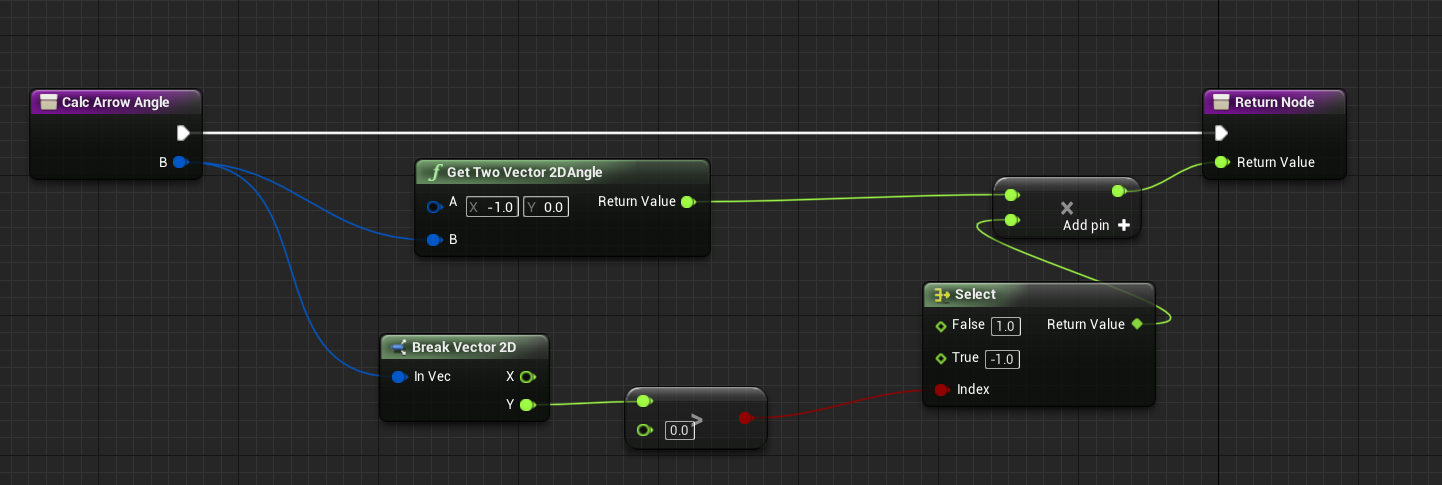##### 按角度旋转向量
• 已知点(x1,y1),绕(x2,y2)旋转θ
• 如果是绕原点旋转，公式可以简化成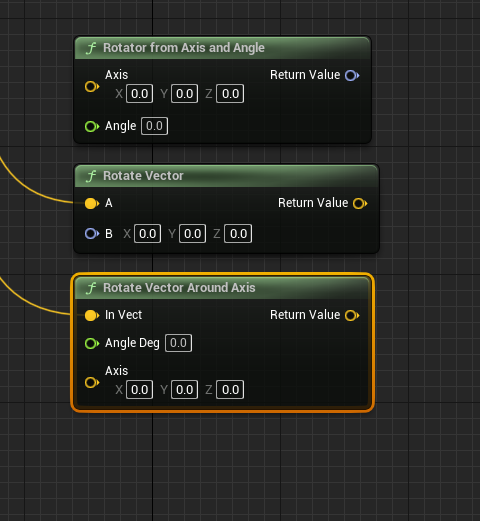## UE中的矩阵变换

### 相对关系

A相对于B的变换信息, 用`TransformA.GetRelativeTransform(TransformB);`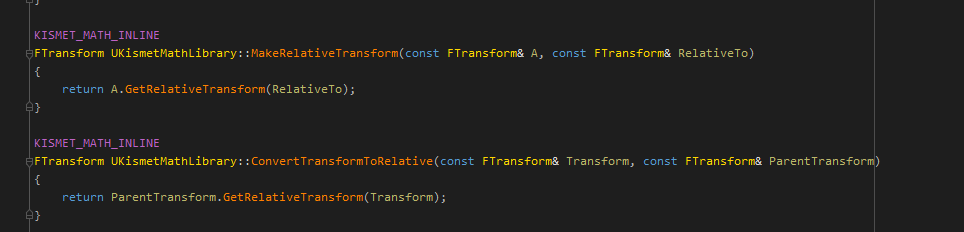`MakeRelativeTransform`的第二个参数输入Parent这个没问题,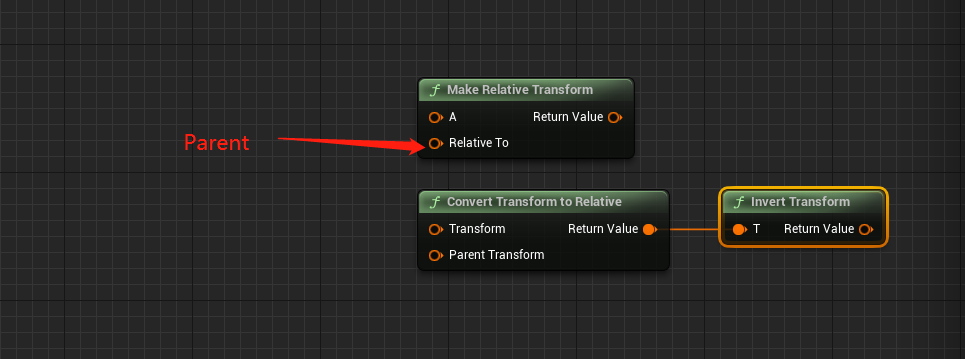### 空间转换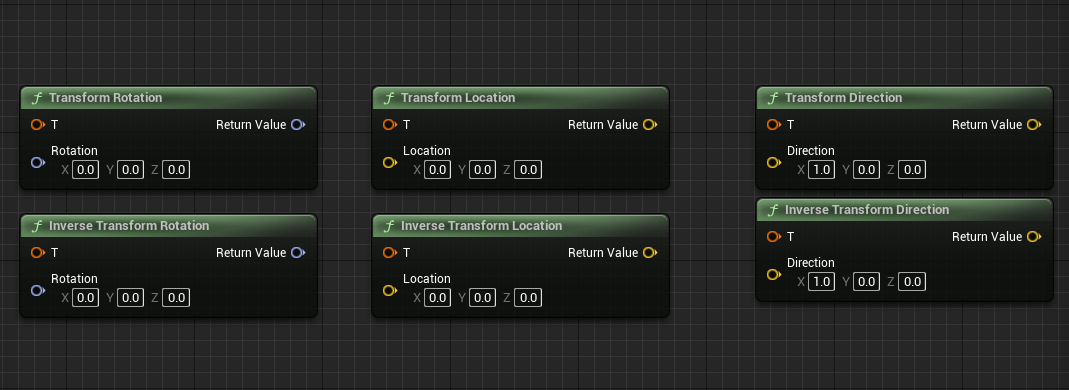### 矩阵乘法

c++中可以直接对Transofomr进行*运算符运算, 在蓝图中包了一层, 使用的是`FTransform UKismetMathLibrary::ComposeTransforms(const FTransform& A, const FTransform& B)`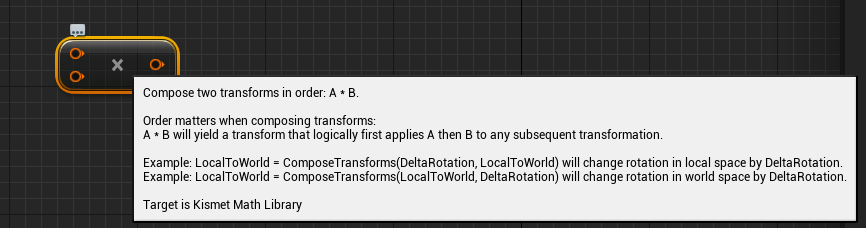1. Actor本地空间进行旋转和偏移,那么使用左乘变换信息, 即 `变换Transform * ActorTransform`
2. 在世界空间中进行变换, 那么可以是变换 `ActorTransform * 变换Transform `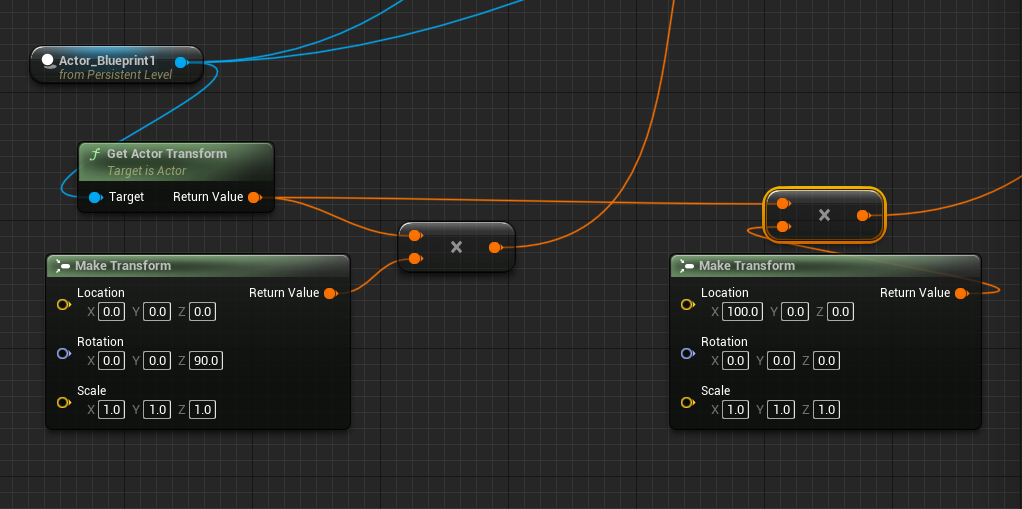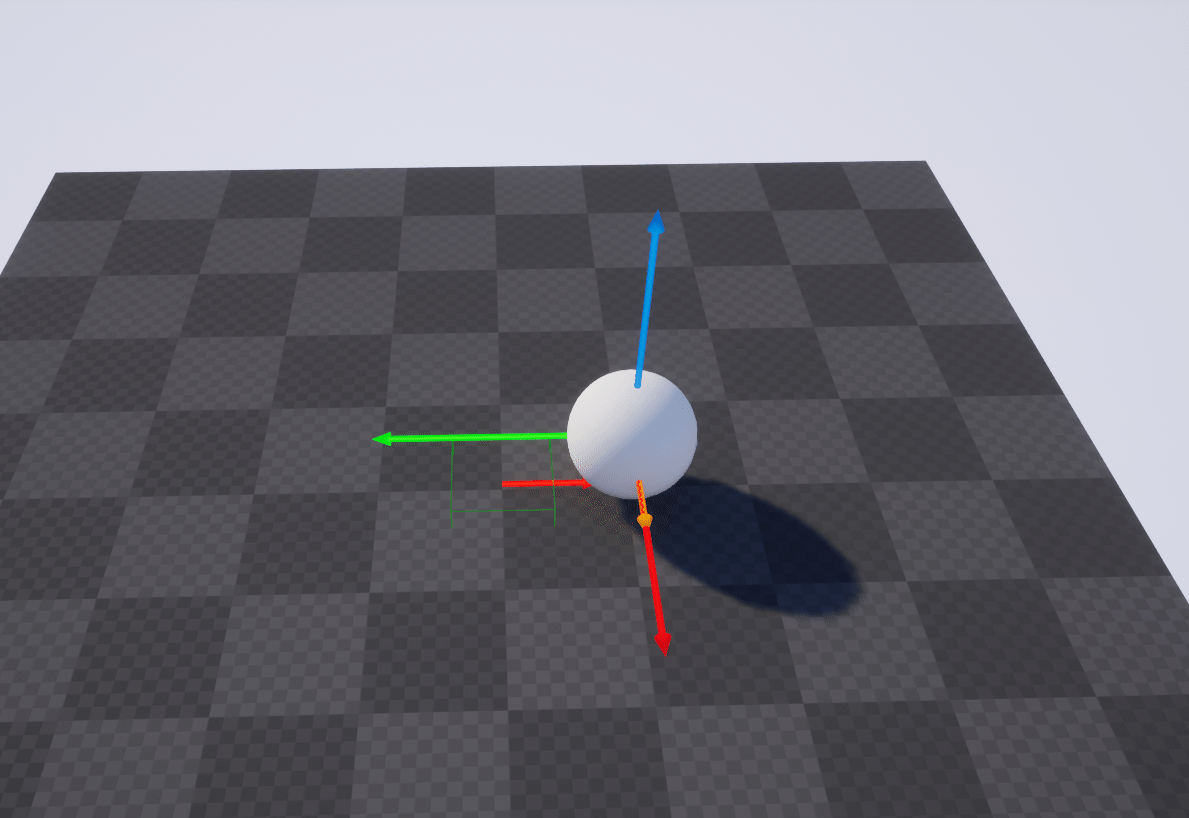## 范数

• 1范数：各个元素的绝对值之和

||X||1=2+3+5+7=17;

• 2范数：各个元素的平方和再开更号

||X||2=(2x2+3x3+5x5+7x7)1/2=9.3274

• p范数：各个向量元素绝对值p次方和的1/p次方
• 无穷范数：各个元素绝对值最大的哪个元素的绝对值微信支付宝